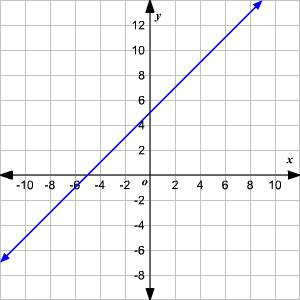# Comparing Functions

There are many ways to represent functions. For example, a function can be represented with an input-output table , with a graph, and with an equation.

Sometimes a problem asks us to compare two functions which are represented in different ways. For example, you might be given a table and a graph, and asked which function is greater for a particular value, or which function increases faster.

Example :

Two functions are represented in different ways.

Function $1$ : The input-output table shows the $x$ - and $y$ -values of a quadratic function .

 $x$ $y$ $0$ $0$ $1$ $1$ $2$ $4$ $3$ $9$ $4$ $16$ $6$ $36$ $8$ $64$

Function $2$ : The graph of a linear function is shown.From the two functions, which function grows faster for large positive values of $x$ ?

In the graph, the $y$ -intercept is $5$ and the slope is $1$ . So, for $x=0$ , the function shown in the graph has a greater value. Also, since the slope is positive, it's increasing.

However, if you look at the values in the table, you will see that the $y$ -values are equal to the square of $x$ . These values will have a faster-than-linear rate of growth.

For example, for the function in the table, when $x=8$ , $y=64$ . You can see in the graph that the line is not yet that high when $x=8$ .